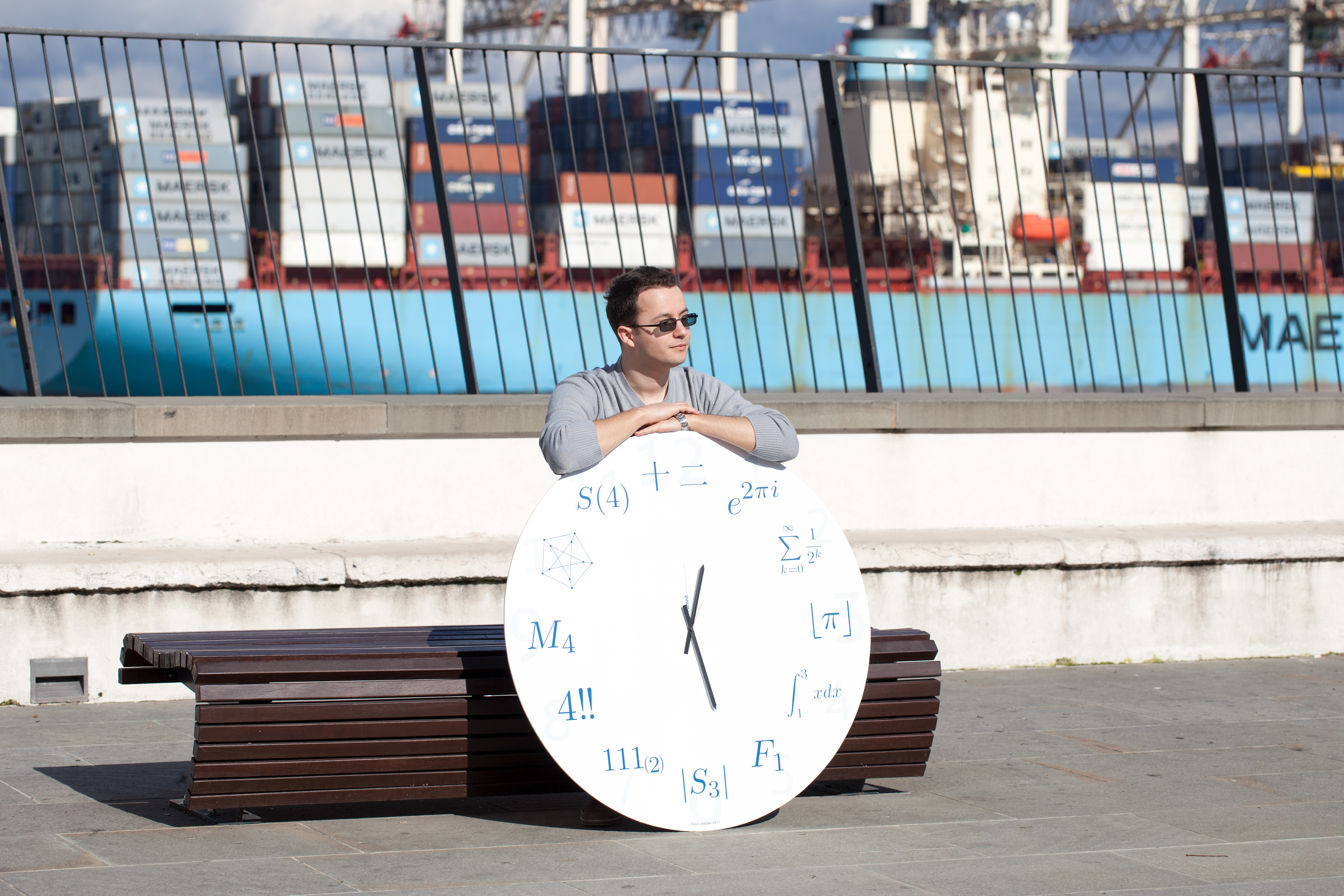Univerza na Primorskem Fakulteta za matematiko, naravoslovje in informacijske tehnologijeSI | EN

# 2013 PhD Summer School in Discrete Mathematics - Short Presentations

natisni

### p, li { white-space: pre-wrap; } Eigenvalues and eigenvectors of finite n-Cayley digraphs over arbitrary groupsMajid Arezoomand, Isfahan University of Technology, Iran

A digraph Γ is called n-Cayley digraph over a group G, if there exists a semiregular subgroup RG of Aut(Γ) isomorphic to G with n orbits. In recent years, the spectrum of n-Cayley graphs in some special cases are computed. For example, the eigenvalues of 2-Cayley graphs and also eigenvalues and eigenvectors of n-Cayley graphs over finite abelian groups are computed in  and , respectively. In this lecture, we extend these results over arbitrary finite groups. (Joint work with Bijan Taeri.)
References:
 Gao, X. and Luo, Y. The spectrum of semi-Cayley graphs over abelian groups, Linear Algebra Appl., 432 (2010) 2974-2983. graphs, (2002). Manuscript for a seminar given at Helsinki University of Technology.
 Kovacs, I., Malnič, A., Marušič, D., and Miklavič, Š. Transitive group actions: (im)primitivity and semiregular subgroups, http://arxiv.org/abs/math/0701686, (2007).
 Arezoomand M., and Taeri. B, On the characteristic polynomial on n-Cayley digraphs, submitted.

### On bounds on the clique and the chromatic number of a vertex transitive graphIgor Đukanović, University of Maribor, Slovenia

László Lovász introduced $\theta$ number sandwiched between the clique and the chromatic number of a graph. It has numerous strengthenings computable in polynomial time toward either number. We apply automorphism group of a graph to dramatically reduce the computational complexity, and generalize well-known equality on vertex transitive graphs $\theta(G) \theta(\bar G)= |V|$ to new strengthened bounds.

### A connection between graph theory and number theoryHau-wen Huang, National Center for Theoretical Sciences, Taiwan

In this talk I will give a brief introduction to the fundamental theorem of graph-theoretical Galois theory, which was first discovered by the German mathematician Reidemeister in 1920's as far as I know. I will also present some recent works joint with Wen-Ching Winnie Li.

### Efficient domination in cubic vertex-transitive graphs on $2^n$ verticesMartin Knor, Slovak University of Technology in Bratislava, Slovakia

A vertex of a graph $G$ dominates itself and all its neighbours. A set $S$, subset of the vertex set of $G$, dominates $G$ efficiently if every vertex of $G$ is dominated by exactly one vertex of $S$. Finally, a Mobius ladder $M_n$ is a cubic graph obtained from the cycle on $2n$ vertices by adding a perfect matching connecting pairs of opposite vertices. Using an algebraic approach based on lifts we prove that, a connected vertex-transitive cubic graph $G$ on $2^m$ vertices does not admit efficient dominating set if and only if $m\ge 3$ and $G$ is isomorphic to the Mobius ladder $M_{2^{m-1}}$. (Joint work with Primož Potočnik.)

### Small subgraphs of Moore graph of degree 57 and its automorphism groupKristína Kováčiková, Comenius University, Slovakia

Let us consider a k-regular graph on n vertices which satisfies two extra conditions. First, there is no triangle in this graph. Second, any two non-adjacent vertices have exactly one common neighbour. Such a graph is called Moore graph of diameter 2. It is well known that there are only 4 feasible values for degree of Moore graph HS, namely 2, 3, 7 and 57. Unlike the first three cases, the existence and uniqueness of the last Moore graph is still an open problem. We study the numbers of occurrences of small graphs as induced subgraphs in this last possible Moore graph. During the talk we want to present our results for several small graphs which are interesting for our purposes in a particular way. We demonstrate an application of these results on automorphisms of order 7 of the examined graph.

### An Action of the Tetrahedron Algebra on the Standard Module of Hamming Graphs and Doob GraphsArlene A. Pascasio, De La Salle University, Manila, Philippines

We show that there exists a tetrahedron algebra module structure on the standard module of Hamming graphs and Doob graphs. To do this, we use a result of Brian Hartwig on tridiagonal pairs of Krawtchouk type. This is joint work with John Vincent S. Morales.

### Leonard pairs and the $q$-Tetrahedron algebraPaul Terwilliger, U. Wisconsin Madison, USA

A Leonard pair is a pair of diagonalizable linear transformations of a finite-dimensional vector space, each of which acts in an irreducible tridiagonal fashion on an eigenbasis for the other one. The Leonard pairs are classified up to isomorphism, and correspond to the orthogonal polynomials from the terminating branch of the Askey scheme. The most general polynomials in this branch are the $q$-Racah polynomials. The $q$-Tetrahedron algebra was introduced in 2006. In this talk we show that each Leonard pair of $q$-Racah type gives a module for the $q$-Tetrahedron algebra. We discuss how this module illuminates the structure of the Leonard pair.